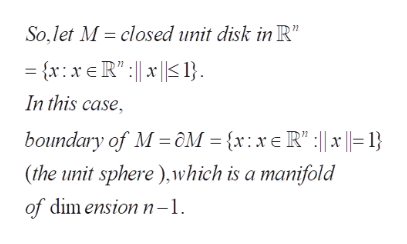# Suppose M is an n-manifold with boundary. Show that ∂M with the subspace topology is an n − 1-manifold (without boundary). You may use that interior(M) ∩ ∂M = ∅. (∂M is the boundary of M).

Question
1 views

Suppose M is an n-manifold with boundary. Show that ∂M with the subspace topology is an n − 1-manifold (without boundary). You may use that interior(M) ∩ ∂M = ∅. (∂M is the boundary of M).

check_circle

Step 1

To prove that the boundary of a n-manifold is a (n-1) manifold without boundary

Step 2

As the question is purely local , we may take the n-manifold M to be the closed unit disk in R^n.

Step 3

The unit sphere is a manifold (without boundary): we can use the atlas provided by the two open sets after removing t...help_outlineImage TranscriptioncloseSo,let M closed unit disk in R" {x: xeR":1} In this case , boundary of M ôM = {x: x e R" :|| |= 1} (fhe unit sphere), which is a manifold of dim ension n-1 fullscreen

### Want to see the full answer?

See Solution

#### Want to see this answer and more?

Solutions are written by subject experts who are available 24/7. Questions are typically answered within 1 hour.*

See Solution
*Response times may vary by subject and question.
Tagged in

### Math